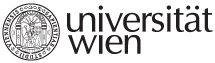NAME OF THE COURSE

INSTRUCTOR AND COURSE CONTENT

ECTS

Quantitative Methods

Professor Ksenija Dumičić, Ph.D.  kdumicic@rfzg.hr

Professor Wolfgang Karl Härdle, Ph.D.

Assistant Professor Krunoslav Puljić, Ph.D.

Course content:

INTRODUCTION. Research process and statistical designs for data collection: experimental design; design of controlled investigations; survey design. Univariate analysis. Variance and data distributions. Types of multivariate methods of analysis and data interrelationships. Software in statistical practice.

## DATA PREPARATION AND INVESTIGATION FOR MULTIVARIATE ANALYSIS. Choosing the technique. Graphical methods of data investigation in multivariate analysis. Identification and estimation of missing data: determining the causes and nature,  the size, diagnosis of randomness, choosing the method of problem elimination. Outliers and detection of outliers.

MULTIPLE REGRESSION. Classical linear regression model. Basic assumptions for the application of the multiple linear regression model. Parameter estimation. Hypothesis testing. Regression diagnostics. Interpretation and validation of results. Forecasting based on regression model.

#### DISCRIMINANT ANALYSIS. Discriminant analysis model. Assumptions for the application of discriminant analysis. Discriminant function. Deriving discriminant function. Direct discriminant analysis. Stepwise discriminant analysis. Interpretation and validation of results.

Statistical softwares in THE APPLICATION OF  multivariate analysis METHODS. CASE ANALYSIS.

INTRODUCTION TO LINEAR AND NON-LINEAR PROGRAMMING

Introduction. Classification of mathematical programming problems. Formulation of some problems in economics as linear and nonlinear programming problems. Linear programming. Simplex method. Duality and its economic interpretation. Sensitivity analysis. Transportation problem. Unconstrained optimization. Constrained optimization with equality constraints. Lagrange multipliers. Nonlinear programming.  Karush-Kuhn-Tucker optimality conditions. Convex programming. Quadratic programming and its applications. Selected numerical methods for solving unconstrained and constrained  optimization problems.

Solving optimization problems using LINDO and LINGO software. Interpretation of results.

5

Methodology of research and qualitative methods

Course content:

Ontological and epistemological basics of scientific theory and scientific research; Methodological monism and pluralism. Business and economic research in a broader scientific context; Conceptual foundations of research (concepts, theories and models; formulation and explanation of research topics and problems). Research approaches, strategies and planning. Measuring of economic variables (levels, scopes and potentials, reliability and validity). Data typology. Searching secondary sources (sources, searching and evaluation techniques). Primary sources (statistical data bases, statistical reports, experimental studies). Data analysis (inductive and deductive methods of qualitative analysis); methodological triangulation; preparation of research reports (written and oral presentations of research papers).

5

Microeconomic analysis

Professor Jasminka Šohinger, Ph.D. (jsohinger@efzg.hr)

Professor Krešimir Žigić, Ph.D.

Associate Professor Ilko Vrankić, Ph.D.

Course content:

INTRODUCTION. Main categories: economic subjects, behavior, institutions, equilibrium.

INDIVIDUAL AND SOCIAL CHOICE.

Consumer preferences. Preference relation. Rationality axioms. Utility function. Consumer choice. Individual demand. Revealed preference. Aggregate demand. Choice under uncertainty. Von Neumann-Morgenstern expected utility. Dynamic choice. Social choice and efficiency. Arrow impossibility theorem.

DECISION MAKING IN PRODUCTION: Technology. Profit function. Derived demand. Cost function. Duality in production. Efficiency in production. The nonsubstitution theorem. Competitive firm. Monopoly. Imperfect competition.

MARKET EQUILIBRIUM AND MARKET FAILURES: Partial equilibrium.  General equilibrium. The existence of general equilibrium. Fixed point theorems. Stability of equilibrium. Efficiency of equilibrium. Welfare economics. The first theorem of welfare economics. The second theorem of welfare economics. Market power. Natural monopoly. Externalities and public goods.

NONCOOPERATIVE GAME THEORY.

Strategic interactions. Extensive form of games. Games in normal or strategic form. Mixed strategies. Kuhn theorem. Nash equilibrium. Equilibrium in mixed strategies.

TOPICS IN THE ECONOMICS OF INFORMATION.  Assymetry of information. Signalling. Moral hazard. Adverse selection. The principal-agent problem.

5

Philosophy of science and research ethics

Associate Profesor D. Lauc, Ph.D. (dlauc@ffzg.hr)

Associate Profesor V. Dorfler, Ph.D.

Course content:

Selected chapters of logic relevant for philosophy of science: Structure of traditional logic – science of elements and science of method. Concepts, propositions and arguments. Definition and division. Deductive and inductive inference. Language and structure of contemporary deductive logic. Deductive systems. The characterization problem. Completeness and decidability. Fundamental notions and methods of decidability theory. Traditional inductive method.

Problem of induction: philosophical problem of induction; justification of induction and new problems, probability and induction, philosophy of probability; inductive logic; Bayesianism and its  problems.

Demarcation of science and pseudoscience; verification and falsification; natural and social sciences; problems of falsification.

Problem of scientific method: logic of discovery and logic of explanation; inductive-deductive and hypothetico-deductive model. For and against method.

Scientific explanation: Hempel’s model; refutation and improvement of models; scientific explanation and probability; unity and economy of science; problem of explanation in social sciences.
Structure of science;  logical reconstruction of science; natural laws; scientific laws and regularities; laws and necessity; laws and social sciences; scientific theories; the notion of scientific paradigm.
The development and the unity of science: cumulative models; the notion of scientific revolutions; Kuhn, sociology of science and science wars;
The question of unity of science, physicalism and reductionism, philosophy of social sciences.
Research ethics in the context of philosophy of science. Values and objectivity. Equal opportunities and inverse discrimination; Should society be protected from science?

5

University of Vienna | Universitätsring 1 | 1010 Vienna | T +43-1-4277-0